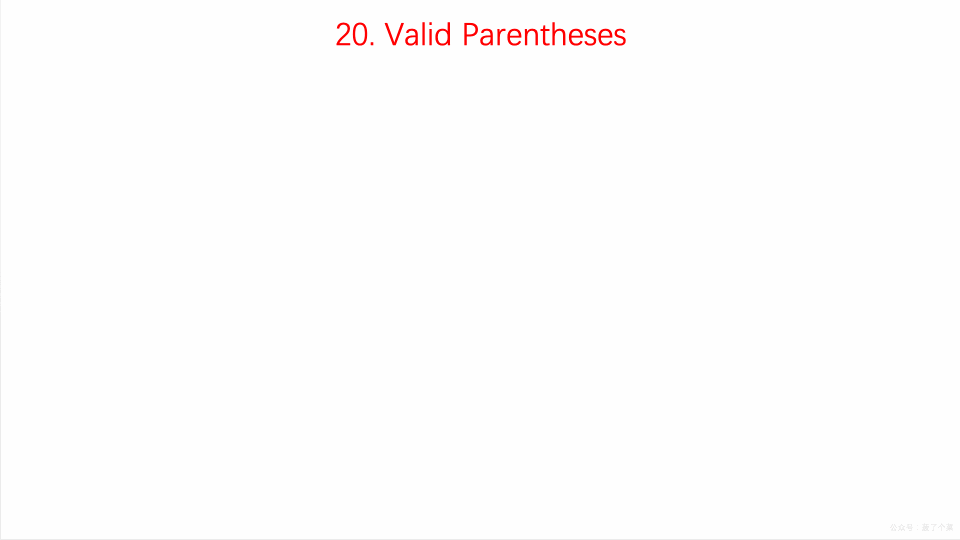# LeetCode 第 20 号问题：有效的括号

### 题目描述

1. 左括号必须用相同类型的右括号闭合。
2. 左括号必须以正确的顺序闭合。

``````输入: "()"

``````

``````输入: "()[]{}"

``````

``````输入: "(]"

``````

``````输入: "([)]"

``````

``````输入: "{[]}"

``````

### 题目解析

• 遍历输入字符串
• 如果当前字符为左半边括号时，则将其压入栈中
• 如果遇到右半边括号时，分类讨论：
• 1）如栈不为空且为对应的左半边括号，则取出栈顶元素，继续循环
• 2）若此时栈为空，则直接返回false
• 3）若不为对应的左半边括号，反之返回false

### 动画描述### 代码实现

``````class Solution {
public:
bool isValid(string s) {

stack<char> stack;
for( int i = 0 ; i < s.size() ; i ++ )
if( s[i] == '(' || s[i] == '{' || s[i] == '[')
stack.push(s[i]);
else{

if( stack.size() == 0 )
return false;

char c = stack.top();
stack.pop();

char match;
if( s[i] == ')' ){
match = '(';
}
else if( s[i] == ']' ){
match = '[';
}
else{
match = '{';
}

if(c != match)  return false;
}

if( stack.size() != 0 )
return false;

return true;
}
};
``````